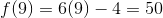# High School Math : Understanding Functional Notations

## Example Questions

### Example Question #1 : Functions And Graphs

Which analysis can be performed to determine if an equation is a function?

Calculating domain and range

Calculating zeroes

Vertical line test

Horizontal line test

Vertical line test

Explanation:

The vertical line test can be used to determine if an equation is a function. In order to be a function, there must only be one(or) value for each value of. The vertical line test determines how many(or) values are present for each value of. If a single vertical line passes through the graph of an equation more than once, it is not a function. If it passes through exactly once or not at all, then the equation is a function.

The horizontal line test can be used to determine if a function is one-to-one, that is, if only onevalue exists for each(or) value. Calculating zeroes, domain, and range can be useful for graphing an equation, but they do not tell if it is a function.

Example of a function: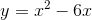Example of an equation that is not a function: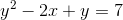### Example Question #2 : Functions And Graphs

Let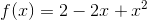and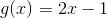. What is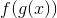?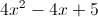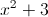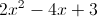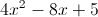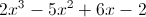Explanation:

THe notationis a composite function, which means we put the inside function g(x) into the outside function f(x). Essentially, we look at the original expression for f(x) and replace each x with the value of g(x).

The original expression for f(x) is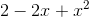. We will take each x and substitute in the value of g(x), which is 2x-1.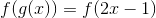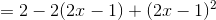We will now distribute the -2 to the 2x - 1.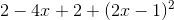We must FOIL the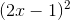term, because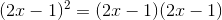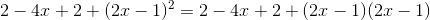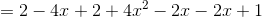Now we collect like terms. Combine the terms with just an x.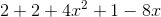Combine constants.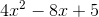The answer is.

### Example Question #1 : Understanding Functional Notations

If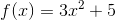and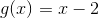, what is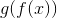?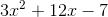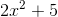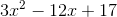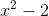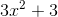Explanation:meansgets plugged into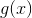.

Thus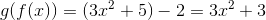.

### Example Question #2 : Understanding Functional Notations

Let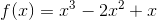and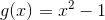.  What is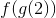?Explanation:

Calculate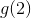and plug it into.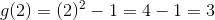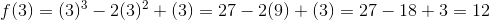### Example Question #3 : Function Notation

Evaluate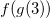if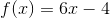and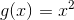.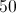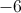Undefined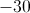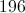Explanation:This expression is the same as saying "take the answer of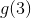and plug it into."

First, we need to find. We do this by pluggingin forin.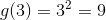Now we take this answer and plug it into.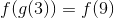We can find the value of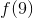by replacingwith.